# Math in Focus Grade 7 Chapter 3 Lesson 3.3 Answer Key Simplifying Algebraic Expressions

Go through the Math in Focus Grade 7 Workbook Answer Key Chapter 3 Lesson 3.3 Simplifying Algebraic Expressions to finish your assignments.

## Math in Focus Grade 7 Course 2 A Chapter 3 Lesson 3.3 Answer Key Simplifying Algebraic Expressions

### Math in Focus Grade 7 Chapter 3 Lesson 3.3 Guided Practice Answer Key

Complete.
Question 1.
1.5t + 4t+ 3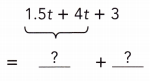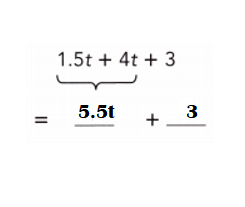Explanation:
1.5t + 4t+ 3
= 5.5t + 3.

Question 2.
1.8m – 0.9m + 2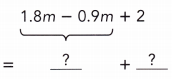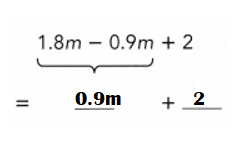Explanation:
1.8m – 0.9m + 2
= 0.9m + 2.

Copy and complete to simplify each expression.
Question 3.
$$\frac{2}{3}$$x – $$\frac{1}{6}$$x + 1 + 6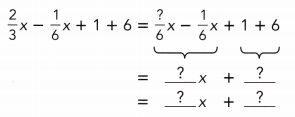$$\frac{2}{3}$$x – $$\frac{1}{6}$$x + 1 + 6 = $$\frac{1}{2}$$x + 7.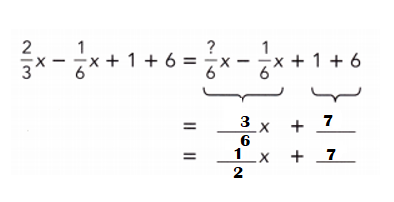Explanation:
$$\frac{2}{3}$$x – $$\frac{1}{6}$$x + 1 + 6
= $$\frac{2}{3}$$x – $$\frac{1}{6}$$x  + 7
LCD of 3 n 6 = 6.
= [(2 × 2)x – (1 × 1)x] ÷ 6 + 7
= (4x – x) ÷ 6 + 7
= 3x ÷ 6 + 7
= x ÷ 2 + 7.

Question 4.
$$\frac{1}{3}$$d + $$\frac{7}{12}$$d – 5 – 1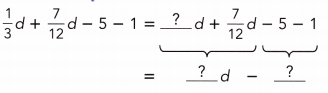$$\frac{1}{3}$$d + $$\frac{7}{12}$$d – 5 – 1 = $$\frac{11}{12}$$d – 4.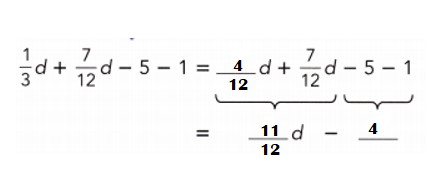Explanation:
$$\frac{1}{3}$$d + $$\frac{7}{12}$$d – 5 – 1
= $$\frac{1}{3}$$d + $$\frac{7}{12}$$d – 4
LCD of 3 n 12 = 12.
= [(1 × 4)d + (7 × 1)d] ÷ 12 – 4
= (4d + 7d) ÷ 12 – 4
= 11d ÷ 12 – 4 or $$\frac{11}{12}$$d – 4.

Question 5.
$$\frac{1}{2}$$k – $$\frac{2}{5}$$k + 8 – 3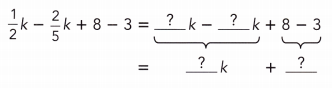$$\frac{1}{2}$$k – $$\frac{2}{5}$$k + 8 – 3 = $$\frac{1}{10}$$k + 5.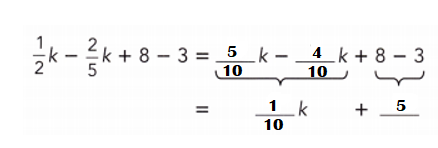Explanation:
$$\frac{1}{2}$$k – $$\frac{2}{5}$$k + 8 – 3
= $$\frac{1}{2}$$k – $$\frac{2}{5}$$k + 5
LCD of 2 n 5 = 10.
= [(1 × 5)k – (2 × 2) k] ÷ 10 + 5
= (5k – 4k) ÷ 10 + 5
= k ÷ 10 + 5 or $$\frac{1}{10}$$k + 5.

Copy and complete to simplify each expression.
Question 6.
0.5k + 0.3k + 1.2k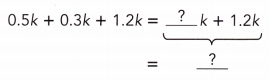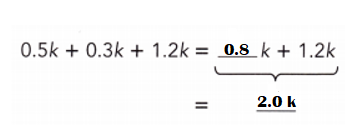Explanation:
0.5k + 0.3k + 1.2k
= 0.8k + 1.2k
= 2.0k.

Question 7.
$$\frac{1}{2}$$p + $$\frac{1}{2}$$p – $$\frac{1}{2}$$p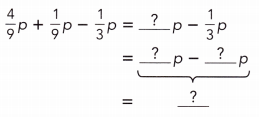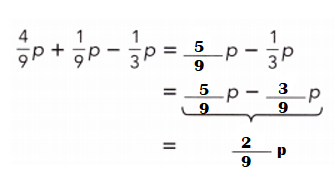Explanation:
$$\frac{1}{2}$$p + $$\frac{1}{2}$$p – $$\frac{1}{2}$$p
= (1 + 1)p ÷ 2 – $$\frac{1}{2}$$p
= 2p ÷ 2 –  $$\frac{1}{2}$$p
= p ÷ 1 – $$\frac{1}{2}$$p
= (2p – p) ÷ 2
= p ÷ 2 or – $$\frac{1}{2}$$p.

Complete to simplify each expression.
Question 8.
4a + 5 + a + 7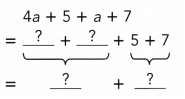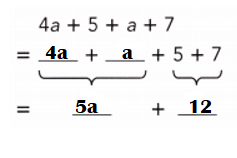Explanation:
4a + 5 + a + 7
= 4a + a + 7 + 5
= 5a + 7 + 5
= 5a + 12.

Question 9.
$$\frac{3}{7}$$x + $$\frac{3}{5}$$ – $$\frac{2}{7}$$x – $$\frac{2}{5}$$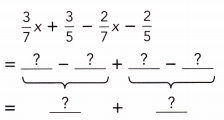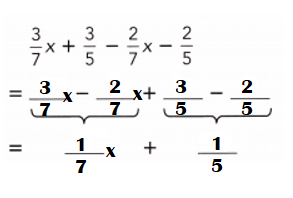Explanation:
$$\frac{3}{7}$$x + $$\frac{3}{5}$$ – $$\frac{2}{7}$$x – $$\frac{2}{5}$$
= $$\frac{3}{7}$$x  – $$\frac{2}{7}$$x  + $$\frac{3}{5}$$ – $$\frac{2}{5}$$
= [(3 – 2)x ÷ 7] + $$\frac{3}{5}$$ – $$\frac{2}{5}$$
= $$\frac{1}{7}$$x + $$\frac{3}{5}$$ – $$\frac{2}{5}$$
= $$\frac{1}{7}$$x + [(3 – 2) ÷ 5]
= $$\frac{1}{7}$$x + $$\frac{1}{5}$$.

Copy and complete to simplify each expression.
Question 10.
6a + 9b + a + b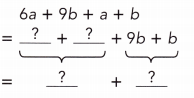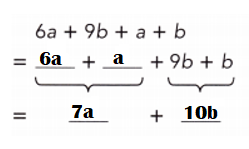Explanation:
6a + 9b + a + b
= 6a + a + 9b + b
= 7a + 9b + b
= 7a + 10b.

Question 11.
3x + 2y – 2x – 3y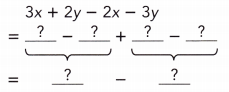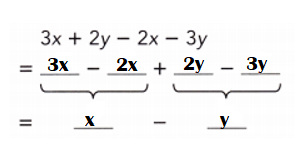Explanation:
3x + 2y – 2x – 3y
= 3x – 2x – 3y + 2y
= x – 3y + 2y
= x – y.

### Math in Focus Course 2A Practice 3.3 Answer Key

Simplify each expression with one variable.
Question 1.
0.3x + 0.6x + 3
0.3x + 0.6x + 3 = 0.9x + 3.

Explanation:
0.3x + 0.6x + 3
= 0.9x + 3.

Question 2.
$$\frac{2}{7}$$x + $$\frac{3}{7}$$x + 4
$$\frac{2}{7}$$x + $$\frac{3}{7}$$x + 4 = $$\frac{5}{7}$$x + 4.

Explanation:
$$\frac{2}{7}$$x + $$\frac{3}{7}$$x + 4
= (2 + 3)x ÷ 7 + 4
= 5x ÷ 7 + 4
= $$\frac{5}{7}$$x + 4.

Question 3.
0.8x – 0.2x – 5
0.8x – 0.2x – 5 = 0.6x – 5.

Explanation:
0.8x – 0.2x – 5
= 0.6x – 5.

Question 4.
$$\frac{7}{8}$$x – $$\frac{1}{8}$$x – 5
$$\frac{7}{8}$$x – $$\frac{1}{8}$$x – 5 = $$\frac{3}{4}$$x – 5.

Explanation:
$$\frac{7}{8}$$x – $$\frac{1}{8}$$x – 5
= (7 – 1)x ÷ 8 – 5
= 6x ÷ 8 – 5
= 3x ÷ 4 – 5  or $$\frac{3}{4}$$x – 5.

Simplify each expression with three algebraic terms.
Question 5.
0.3p + 0.2p + 0.4p
0.3p + 0.2p + 0.4p = 0.9p.

Explanation:
0.3p + 0.2p + 0.4p
= 0.5p + 0.4p
= 0.9p.

Question 6.
0.2 + o.8p – 0.6p
0.2 + o.8p – 0.6p = 0.2(1 + p).

Explanation:
0.2 + o.8p – 0.6p
= 0.2 + 0.2p.
= 0.2(1 + p).

Question 7.
$$\frac{1}{3}$$y + $$\frac{1}{6}$$y + $$\frac{5}{12}$$y
$$\frac{1}{3}$$y + $$\frac{1}{6}$$y + $$\frac{5}{12}$$y = $$\frac{2}{3}$$y.

Explanation:
$$\frac{1}{3}$$y + $$\frac{1}{6}$$y + $$\frac{5}{12}$$y
= LCD of 3 n 6 = 6.
= [(2 + 1)y ÷ 6] + $$\frac{5}{12}$$y
= 3y ÷ 6 + $$\frac{5}{12}$$y
= y ÷ 6 + $$\frac{5}{12}$$y
= $$\frac{1}{6}$$y + $$\frac{5}{12}$$y
LCD of 6 n 12 = 12.
= [(1 × 2) + 5]y ÷ 12
= (3 + 5)y ÷ 12
= 8y ÷ 12
= 2y ÷ 3 or $$\frac{2}{3}$$y.

Question 8.
$$\frac{1}{2}$$a + $$\frac{2}{3}$$a – $$\frac{1}{6}$$a
$$\frac{1}{2}$$a + $$\frac{2}{3}$$a – $$\frac{1}{6}$$a = a.

Explanation:
$$\frac{1}{2}$$a + $$\frac{2}{3}$$a – $$\frac{1}{6}$$a
= LCD of 2 n 3 = 6.
= [(1 × 3) + (2 × 2)]a ÷ 6 – $$\frac{1}{6}$$a
= (3 + 4)a ÷ 6 – $$\frac{1}{6}$$a
= $$\frac{7}{6}$$a –  $$\frac{1}{6}$$a
= (7 – 1)a ÷ 6
= 6a ÷ 6
= a ÷ 1 or a.

Simplify each expression with one variable.
Question 9.
7x + 6 + 4x
7x + 6 + 4x = 11x + 6.

Explanation:
7x + 6 + 4x
= 7x + 4x + 6
= 11x + 6.

Question 10.
8y – 4 – 6y
8y – 4 – 6y = 2y – 4.

Explanation:
8y – 4 – 6y
= 8y – 6y – 4
= 2y – 4.

Question 11.
1.8x – 0.6 – 0.7x
1.8x – 0.6 – 0.7x = 1.1x – 0.6.

Explanation:
1.8x – 0.6 – 0.7x
= 1.8x – 0.7x – 0.6
= 1.1x – 0.6.

Question 12.
$$\frac{4}{7}$$x + $$\frac{1}{5}$$ + $$\frac{2}{7}$$x
$$\frac{4}{7}$$x + $$\frac{1}{5}$$ + $$\frac{2}{7}$$x = $$\frac{6}{7}$$x + $$\frac{1}{5}$$.

Explanation:
$$\frac{4}{7}$$x + $$\frac{1}{5}$$ + $$\frac{2}{7}$$x
= $$\frac{4}{7}$$x  + $$\frac{2}{7}$$x + $$\frac{1}{5}$$
= (4 + 2)x ÷ 7 + $$\frac{1}{5}$$
= 6x ÷ 7 + $$\frac{1}{5}$$
= $$\frac{6}{7}$$x + $$\frac{1}{5}$$

Simplify each expression with two variables.
Question 13.
2x + 4x + 7y
2x + 4x + 7y = 6x + 7y.

Explanation:
2x + 4x + 7y
= 6x + 7y.

Question 14.
5x + x + 3y
5x + x + 3y = 6x + 3y.

Explanation:
5x + x + 3y
= 6x + 3y.

Question 15.
9x + 7x + 3y
9x + 7x + 3y = 16x + 3y.

Explanation:
9x + 7x + 3y
= 16x + 3y.

Question 16.
8m – 7m – 6n
8m – 7m – 6n = m – 6n.

Explanation:
8m – 7m – 6n
= m – 6n.

Question 17.
2.3a – 1.8a + 3.5b – 2.7b
2.3a – 1.8a + 3.5b – 2.7b = 0.5a + 0.8b.

Explanation:
2.3a – 1.8a + 3.5b – 2.7b
= 0.5a + 3.5b – 2.7b
= 0.5a + 0.8b.

Question 18.
2.5x + 1.8z + 1.6x + 0.9z
2.5x + 1.8z + 1.6x + 0.9z = 4.1x + 2.7z.

Explanation:
2.5x + 1.8z + 1.6x + 0.9z
= 2.5x + 1.6x + 1.8z + 0.9z
= 4.1x + 2.7z.

Question 19.
$$\frac{3}{5}$$a + $$\frac{2}{5}$$a + $$\frac{1}{6}$$b + $$\frac{1}{6}$$b
$$\frac{3}{5}$$a + $$\frac{2}{5}$$a + $$\frac{1}{6}$$b + $$\frac{1}{6}$$b = a + $$\frac{1}{3}$$b.

Explanation:
$$\frac{3}{5}$$a + $$\frac{2}{5}$$a + $$\frac{1}{6}$$b + $$\frac{1}{6}$$b
= [(3 + 2)a ÷ 5] + $$\frac{1}{6}$$b + $$\frac{1}{6}$$b
= [5a ÷ 5] + $$\frac{1}{6}$$b + $$\frac{1}{6}$$b
= a +  $$\frac{1}{6}$$b + $$\frac{1}{6}$$b
= a + [(1 + 1)b ÷ 6]
= a + [2b ÷ 6]
= a + $$\frac{1}{3}$$b.

Question 20.
$$\frac{2}{3}$$a – $$\frac{1}{6}$$a + $$\frac{3}{5}$$b – $$\frac{3}{10}$$b
$$\frac{2}{3}$$a – $$\frac{1}{6}$$a + $$\frac{3}{5}$$b – $$\frac{3}{10}$$b = $$\frac{1}{2}$$a  + $$\frac{3}{10}$$b.

Explanation:
$$\frac{2}{3}$$a – $$\frac{1}{6}$$a + $$\frac{3}{5}$$b – $$\frac{3}{10}$$b
LCD of 3 n 6 = 6.
= [[(2 × 2) – (1 × 1)]a ÷ 6] + $$\frac{3}{5}$$b – $$\frac{3}{10}$$b
= [(4 – 1)a ÷ 6] + $$\frac{3}{5}$$b – $$\frac{3}{10}$$b
= (3a ÷ 6) + $$\frac{3}{5}$$b – $$\frac{3}{10}$$b
= $$\frac{1}{2}$$a + $$\frac{3}{5}$$b – $$\frac{3}{10}$$b
LCD of 5 n 10 = 10.
= $$\frac{1}{2}$$a  + [[(3 × 2) – (3 × 1)]b ÷ 10]
= $$\frac{1}{2}$$a + [(6 – 3)b ÷ 10]
= $$\frac{1}{2}$$a + (3b ÷ 10)
= $$\frac{1}{2}$$a  + $$\frac{3}{10}$$b.

Find the perimeter of each figure.
Question 21.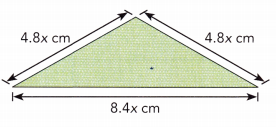Perimeter of triangle = 18cm.

Explanation:
Perimeter of triangle = Side + side + side
= 4.8cm + 4.8cm + 8.4cm
= 9.6cm + 8.4cm
= 18cm.

Question 22.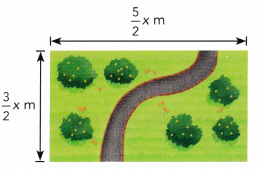Perimeter of rectangle = 8m.

Explanation:
Perimeter of rectangle = 2(Length + Width)
= 2( $$\frac{5}{2}$$m + $$\frac{3}{2}$$m)
= 2[(5 + 3)÷2]m
= 2 (8 ÷ 2)m
= 2 × 4m
= 8m.

Question 23.
Math journal When adding or subtracting algebraic expressions, how do you identify the like terms?